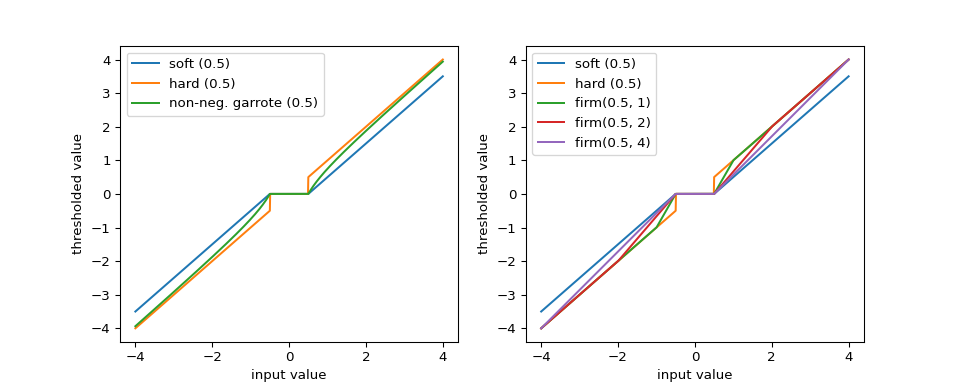# Thresholding functions¶

The thresholding helper module implements the most popular signal thresholding functions.

## Thresholding¶

pywt.threshold(data, value, mode='soft', substitute=0)

Thresholds the input data depending on the mode argument.

In soft thresholding , data values with absolute value less than param are replaced with substitute. Data values with absolute value greater or equal to the thresholding value are shrunk toward zero by value. In other words, the new value is data/np.abs(data) * np.maximum(np.abs(data) - value, 0).

In hard thresholding, the data values where their absolute value is less than the value param are replaced with substitute. Data values with absolute value greater or equal to the thresholding value stay untouched.

garrote corresponds to the Non-negative garrote threshold , . It is intermediate between hard and soft thresholding. It behaves like soft thresholding for small data values and approaches hard thresholding for large data values.

In greater thresholding, the data is replaced with substitute where data is below the thresholding value. Greater data values pass untouched.

In less thresholding, the data is replaced with substitute where data is above the thresholding value. Lesser data values pass untouched.

Both hard and soft thresholding also support complex-valued data.

Parameters: data : array_like Numeric data. value : scalar Thresholding value. mode : {‘soft’, ‘hard’, ‘garrote’, ‘greater’, ‘less’} Decides the type of thresholding to be applied on input data. Default is ‘soft’. substitute : float, optional Substitute value (default: 0). output : array Thresholded array.

References

  D.L. Donoho and I.M. Johnstone. Ideal Spatial Adaptation via Wavelet Shrinkage. Biometrika. Vol. 81, No. 3, pp.425-455, 1994. DOI:10.1093/biomet/81.3.425
  L. Breiman. Better Subset Regression Using the Nonnegative Garrote. Technometrics, Vol. 37, pp. 373-384, 1995. DOI:10.2307/1269730
  H-Y. Gao. Wavelet Shrinkage Denoising Using the Non-Negative Garrote. Journal of Computational and Graphical Statistics Vol. 7, No. 4, pp.469-488. 1998. DOI:10.1080/10618600.1998.10474789

Examples

>>> import numpy as np
>>> import pywt
>>> data = np.linspace(1, 4, 7)
>>> data
array([ 1. ,  1.5,  2. ,  2.5,  3. ,  3.5,  4. ])
>>> pywt.threshold(data, 2, 'soft')
array([ 0. ,  0. ,  0. ,  0.5,  1. ,  1.5,  2. ])
>>> pywt.threshold(data, 2, 'hard')
array([ 0. ,  0. ,  2. ,  2.5,  3. ,  3.5,  4. ])
>>> pywt.threshold(data, 2, 'garrote')
array([ 0.        ,  0.        ,  0.        ,  0.9       ,  1.66666667,
2.35714286,  3.        ])
>>> pywt.threshold(data, 2, 'greater')
array([ 0. ,  0. ,  2. ,  2.5,  3. ,  3.5,  4. ])
>>> pywt.threshold(data, 2, 'less')
array([ 1. ,  1.5,  2. ,  0. ,  0. ,  0. ,  0. ])

pywt.threshold_firm(data, value_low, value_high)

Firm threshold.

The approach is intermediate between soft and hard thresholding . It behaves the same as soft-thresholding for values below value_low and the same as hard-thresholding for values above thresh_high. For intermediate values, the thresholded value is in between that corresponding to soft or hard thresholding.

Parameters: data : array-like The data to threshold. This can be either real or complex-valued. value_low : float Any values smaller then value_low will be set to zero. value_high : float Any values larger than value_high will not be modified. val_new : array-like The values after firm thresholding at the specified thresholds.

Notes

This thresholding technique is also known as semi-soft thresholding .

For each value, x, in data. This function computes:

if np.abs(x) <= value_low:
return 0
elif np.abs(x) > value_high:
return x
elif value_low < np.abs(x) and np.abs(x) <= value_high:
return x * value_high * (1 - value_low/x)/(value_high - value_low)


firm is a continuous function (like soft thresholding), but is unbiased for large values (like hard thresholding).

If value_high == value_low this function becomes hard-thresholding. If value_high is infinity, this function becomes soft-thresholding.

References

  H.-Y. Gao and A.G. Bruce. Waveshrink with firm shrinkage. Statistica Sinica, Vol. 7, pp. 855-874, 1997.
  A. Bruce and H-Y. Gao. WaveShrink: Shrinkage Functions and Thresholds. Proc. SPIE 2569, Wavelet Applications in Signal and Image Processing III, 1995. DOI:10.1117/12.217582

The left panel of the figure below illustrates that non-negative Garotte thresholding is intermediate between soft and hard thresholding. Firm thresholding transitions between soft and hard thresholding behavior. It requires a pair of threshold values that define the width of the transition region.

import numpy as np
import matplotlib.pyplot as plt
import pywt

s = np.linspace(-4, 4, 1000)

s_soft = pywt.threshold(s, value=0.5, mode='soft')
s_hard = pywt.threshold(s, value=0.5, mode='hard')
s_garrote = pywt.threshold(s, value=0.5, mode='garrote')
s_firm1 = pywt.threshold_firm(s, value_low=0.5, value_high=1)
s_firm2 = pywt.threshold_firm(s, value_low=0.5, value_high=2)
s_firm3 = pywt.threshold_firm(s, value_low=0.5, value_high=4)

fig, ax = plt.subplots(1, 2, figsize=(10, 4))
ax.plot(s, s_soft)
ax.plot(s, s_hard)
ax.plot(s, s_garrote)
ax.legend(['soft (0.5)', 'hard (0.5)', 'non-neg. garrote (0.5)'])
ax.set_xlabel('input value')
ax.set_ylabel('thresholded value')

ax.plot(s, s_soft)
ax.plot(s, s_hard)
ax.plot(s, s_firm1)
ax.plot(s, s_firm2)
ax.plot(s, s_firm3)
ax.legend(['soft (0.5)', 'hard (0.5)', 'firm(0.5, 1)', 'firm(0.5, 2)',
'firm(0.5, 4)'])
ax.set_xlabel('input value')
ax.set_ylabel('thresholded value')
plt.show()